• 【机器学习】python使用支持向量机SVM准备： 数据集 导入SVM模块步骤： 1.读取数据集 2.划分训练样本与测试样本 3.训练SVM分类器 4.计算分类准确率 5.绘制图像 关于SVM的原理知识，在【机器学习】支持向量机...
【机器学习】python使用支持向量机SVM准备：        数据集    导入SVM模块步骤：    1.读取数据集    2.划分训练样本与测试样本    3.训练SVM分类器    4.计算分类准确率    5.绘制图像        关于SVM的原理知识，在【机器学习】支持向量机中讲过，欲知详情，可戳：    https://blog.csdn.net/u012679707/article/details/80501358    在Matlab中可以使用工具箱中的svm算法，具体实例在之前的人脸识别matlab实现中讲过，可戳：    https://blog.csdn.net/u012679707/article/details/78152713    因为Python中的sklearn库也集成了SVM算法，所以在Python中一样可以使用支持向量机做分类。    【注意】本文的运行环境是windows+Pycharm+python3.6。    Scikit-Learn库基本实现了所有的机器学习算法，具体使用详见官方文档说明：    http://scikit-learn.org/stable/auto_examples/index.html#support-vector-machines    因为本文是基于sklearn包，所以需先在python中下载sklearn包，网上有很多教程，在此不再叙述。    数据集    本文用的数据集为Iris.data可从UCI数据库中下载，http://archive.ics.uci.edu/ml/datasets/Iris    Iris.data的数据格式如下：共5列，前4列为样本特征，第5列为类别，分别有三种类别Iris-setosa， Iris-versicolor， Iris-virginica。    注意：因为在分类中类别标签必须为数字量，所以应将Iris.data中的第5列的类别（字符串）转换为num.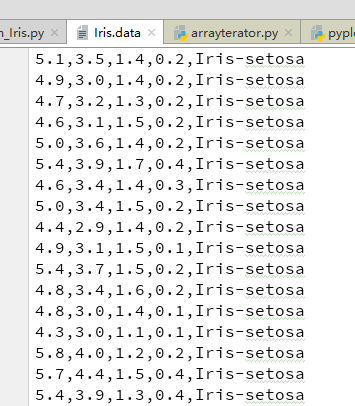导入SVM模块    首先在使用SVM时，需先从sklearn包中导入SVM模块。from sklearn import svm1.读取数据集#1.读取数据集
path='F:/Python_Project/SVM/data/Iris.data'
#converters={4:Iris_label}中“4”指的是第5列：将第5列的str转化为label(number)    定义的转换函数为：可实现将类别Iris-setosa， Iris-versicolor， Iris-virginica映射成 0,1,2。#define converts(字典)
def Iris_label(s):
it={b'Iris-setosa':0, b'Iris-versicolor':1, b'Iris-virginica':2 }
return it[s]    读取文件用的是loadtxt函数，其声明如下：def  loadtxt(fname, dtype=float, comments='#', delimiter=None,converters=None, skiprows=0, usecols=None, unpack=False, ndmin=0)    常用的参数有：        fname: 文件路径，例 path='F:/Python_Project/SVM/data/Iris.data'   dtype:样本的数据类型 例dtype=float          delimiter：分隔符。例 delimiter=','          converters：将数据列与转换函数进行映射的字典。例 converters={4:Iris_label}含义是将第5列的数据对应转换函数进行转换。          usecols：选取数据的列。2.划分训练样本与测试样本#2.划分数据与标签
x,y=np.split(data,indices_or_sections=(4,),axis=1) #x为数据，y为标签
x=x[:,0:2] #为便于后边画图显示，只选取前两维度。若不用画图，可选取前四列x[:,0:4]
train_data,test_data,train_label,test_label =sklearn.model_selection.train_test_split(x,y, random_state=1, train_size=0.6,test_size=0.4)
      1. split(数据，分割位置，轴=1（水平分割） or 0（垂直分割）)。　　2.  sklearn.model_selection.train_test_split随机划分训练集与测试集。train_test_split(train_data,train_label,test_size=数字, random_state=0)　　参数解释：　    　train_data：所要划分的样本特征集　    　train_label：所要划分的样本类别　    　test_size：样本占比，如果是整数的话就是样本的数量.(注意：)                   --  test_size:测试样本占比。 默认情况下，该值设置为0.25。 默认值将在版本0.21中更改。 只有train_size没有指定时，                         它将保持0.25，否则它将补充指定的train_size，例如train_size=0.6,则test_size默认为0.4。                   -- train_size:训练样本占比。　    　random_state：是随机数的种子。　　    随机数种子：其实就是该组随机数的编号，在需要重复试验的时候，保证得到一组一样的随机数。比如你每次都填1，其他参数一样的情况下你得到的随机数组是一样的。但填0或不填，每次都会不一样。随机数的产生取决于种子，随机数和种子之间的关系遵从以下两个规则：种子不同，产生不同的随机数；种子相同，即使实例不同也产生相同的随机数。3.训练SVM分类器#3.训练svm分类器
classifier=svm.SVC(C=2,kernel='rbf',gamma=10,decision_function_shape='ovr') # ovr:一对多策略
classifier.fit(train_data,train_label.ravel()) #ravel函数在降维时默认是行序优先    kernel='linear'时，为线性核，C越大分类效果越好，但有可能会过拟合（defaul C=1）。　 kernel='rbf'时（default），为高斯核，gamma值越小，分类界面越连续；gamma值越大，分类界面越“散”，分类效果越好，但有可能会过拟合。　 decision_function_shape='ovr'时，为one v rest（一对多），即一个类别与其他类别进行划分，　 decision_function_shape='ovo'时，为one v one（一对一），即将类别两两之间进行划分，用二分类的方法模拟多分类的结果。4.计算分类准确率#4.计算svc分类器的准确率
print("训练集：",classifier.score(train_data,train_label))
print("测试集：",classifier.score(test_data,test_label))    结果：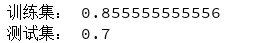还有另一种计算准确率的方法:#也可直接调用accuracy_score方法计算准确率
from sklearn.metrics import accuracy_score
tra_label=classifier.predict(train_data) #训练集的预测标签
tes_label=classifier.predict(test_data) #测试集的预测标签
print("训练集：", accuracy_score(train_label,tra_label) )
print("测试集：", accuracy_score(test_label,tes_label) )    实际上，classifier.score（）内部也是先predict得到tes_label , 然后调用了accuracy_score(test_label,tes_label)方法来计算准确率的。    可以查看一下内部决策函数，返回的是样本到分类超平面的距离，#查看决策函数
print('train_decision_function:',classifier.decision_function(train_data)) # (90,3)
print('predict_result:',classifier.predict(train_data))   （1） 若选用“ovr”(一对多)，则每个样本会产生3个距离值（3为类别种类数）。如下：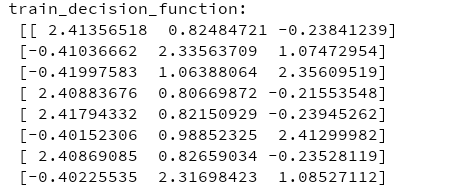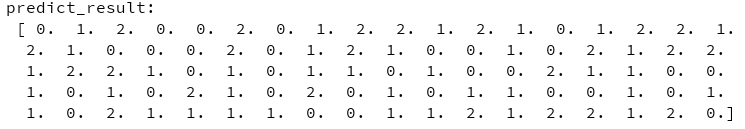（2）若选用“ovo”(一对一)，则每个样本会产生3*（3-1）/2=3 个距离值,即 ：距离值个数=类别数*（类别数-1）/2。结果如下：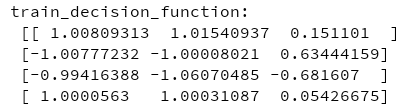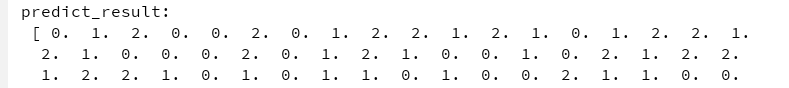5.绘制图像    确定坐标轴范围、字体、背景颜色#5.绘制图形
#确定坐标轴范围
x1_min, x1_max=x[:,0].min(), x[:,0].max() #第0维特征的范围
x2_min, x2_max=x[:,1].min(), x[:,1].max() #第1维特征的范围
x1,x2=np.mgrid[x1_min:x1_max:200j, x2_min:x2_max:200j ] #生成网络采样点
grid_test=np.stack((x1.flat,x2.flat) ,axis=1) #测试点
#指定默认字体
matplotlib.rcParams['font.sans-serif']=['SimHei']
#设置颜色
cm_light=matplotlib.colors.ListedColormap(['#A0FFA0', '#FFA0A0', '#A0A0FF'])
cm_dark=matplotlib.colors.ListedColormap(['g','r','b'] )

grid_hat = classifier.predict(grid_test)       # 预测分类值
grid_hat = grid_hat.reshape(x1.shape)  # 使之与输入的形状相同    这里用到了mgrid()函数，该函数的作用这里简单介绍一下：　　 假设假设目标函数F（x，y）=x+y。x轴范围1~3，y轴范围4~6，当绘制图像时主要分四步进行：　　【step1：x扩展】(朝右扩展)：       [1 1 1]　　 [2 2 2]　　 [3 3 3]　　【step2：y扩展】(朝下扩展)：　　 [4 5 6]　　 [4 5 6]　　 [4 5 6]　　【step3：定位（xi，yi）】：　　 [(1,4) (1,5) (1,6)]　　 [(2,4) (2,5) (2,6)]　　 [(3,4) (3,5) (3,6)]　　【step4：将（xi，yi）代入F(x,y)=x+y】　　因此这里x1, x2 = np.mgrid[x1_min:x1_max:200j, x2_min:x2_max:200j]后的结果为：再通过stack()函数，axis=1，生成测试点绘图：plt.pcolormesh(x1, x2, grid_hat, cmap=cm_light)     # 预测值的显示
plt.scatter(x[:, 0], x[:, 1], c=y[:,0], s=30,cmap=cm_dark)  # 样本
plt.scatter(test_data[:,0],test_data[:,1], c=test_label[:,0],s=30,edgecolors='k', zorder=2,cmap=cm_dark) #圈中测试集样本点
plt.xlabel('花萼长度', fontsize=13)
plt.ylabel('花萼宽度', fontsize=13)
plt.xlim(x1_min,x1_max)
plt.ylim(x2_min,x2_max)
plt.title('鸢尾花SVM二特征分类')
plt.show()          pcolormesh(x,y,z,cmap)这里参数代入x1，x2，grid_hat，cmap=cm_light绘制的是背景。　　    scatter中edgecolors是指描绘点的边缘色彩，s指描绘点的大小，cmap指点的颜色。　    　xlim指图的边界。     pcolormesh(x,y,z,cmap)绘制的背景如下：即将坐标系下的点都进行一个分类。plt.pcolormesh(x1, x2, grid_hat, cmap=cm_light)     # 预测值的显示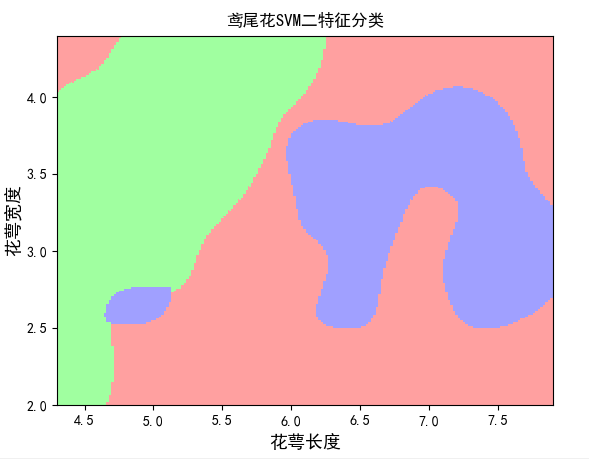所有样本点的分类结果：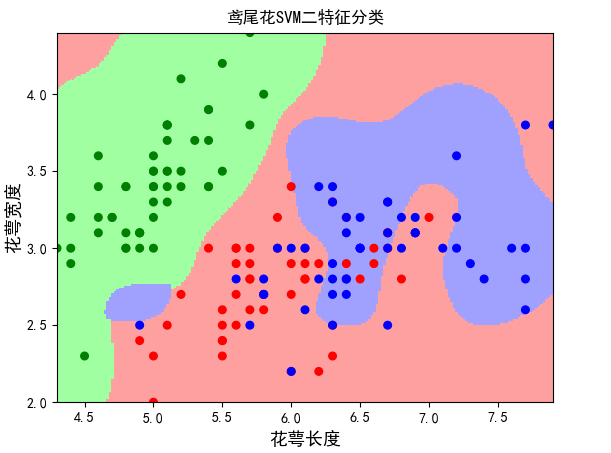将测试点从中圈出来：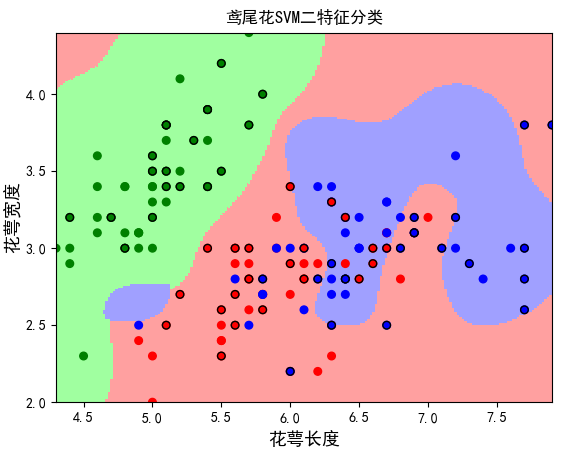完整代码如下：# -*- coding:utf-8 -*-
"""
@author:Lisa
@file:svm_Iris.py
@func:Use SVM to achieve Iris flower classification
@time:2018/5/30 0030上午 9:58
"""
from sklearn import svm
import numpy as np
import matplotlib.pyplot as plt
import matplotlib
import sklearn
from sklearn.model_selection import train_test_split

#define converts(字典)
def Iris_label(s):
it={b'Iris-setosa':0, b'Iris-versicolor':1, b'Iris-virginica':2 }
return it[s]

#1.读取数据集
path='F:/Python_Project/SVM/data/Iris.data'
#converters={4:Iris_label}中“4”指的是第5列：将第5列的str转化为label(number)
#print(data.shape)

#2.划分数据与标签
x,y=np.split(data,indices_or_sections=(4,),axis=1) #x为数据，y为标签
x=x[:,0:2]
train_data,test_data,train_label,test_label =train_test_split(x,y, random_state=1, train_size=0.6,test_size=0.4) #sklearn.model_selection.
#print(train_data.shape)

#3.训练svm分类器
classifier=svm.SVC(C=2,kernel='rbf',gamma=10,decision_function_shape='ovo') # ovr:一对多策略
classifier.fit(train_data,train_label.ravel()) #ravel函数在降维时默认是行序优先

#4.计算svc分类器的准确率
print("训练集：",classifier.score(train_data,train_label))
print("测试集：",classifier.score(test_data,test_label))

#也可直接调用accuracy_score方法计算准确率
from sklearn.metrics import accuracy_score
tra_label=classifier.predict(train_data) #训练集的预测标签
tes_label=classifier.predict(test_data) #测试集的预测标签
print("训练集：", accuracy_score(train_label,tra_label) )
print("测试集：", accuracy_score(test_label,tes_label) )

#查看决策函数
print('train_decision_function:\n',classifier.decision_function(train_data)) # (90,3)
print('predict_result:\n',classifier.predict(train_data))

#5.绘制图形
#确定坐标轴范围
x1_min, x1_max=x[:,0].min(), x[:,0].max() #第0维特征的范围
x2_min, x2_max=x[:,1].min(), x[:,1].max() #第1维特征的范围
x1,x2=np.mgrid[x1_min:x1_max:200j, x2_min:x2_max:200j ] #生成网络采样点
grid_test=np.stack((x1.flat,x2.flat) ,axis=1) #测试点
#指定默认字体
matplotlib.rcParams['font.sans-serif']=['SimHei']
#设置颜色
cm_light=matplotlib.colors.ListedColormap(['#A0FFA0', '#FFA0A0', '#A0A0FF'])
cm_dark=matplotlib.colors.ListedColormap(['g','r','b'] )

grid_hat = classifier.predict(grid_test)       # 预测分类值
grid_hat = grid_hat.reshape(x1.shape)  # 使之与输入的形状相同

plt.pcolormesh(x1, x2, grid_hat, cmap=cm_light)     # 预测值的显示
plt.scatter(x[:, 0], x[:, 1], c=y[:,0], s=30,cmap=cm_dark)  # 样本
plt.scatter(test_data[:,0],test_data[:,1], c=test_label[:,0],s=30,edgecolors='k', zorder=2,cmap=cm_dark) #圈中测试集样本点
plt.xlabel('花萼长度', fontsize=13)
plt.ylabel('花萼宽度', fontsize=13)
plt.xlim(x1_min,x1_max)
plt.ylim(x2_min,x2_max)
plt.title('鸢尾花SVM二特征分类')
plt.show() -------------------------------------------         END      -------------------------------------参考：Python中的支持向量机SVM的使用（有实例） http://www.cnblogs.com/luyaoblog/p/6775342.html（非常好）
展开全文• python数据挖掘系列教程 线性函数、线性回归、线性分类 参考：http://blog.csdn.net/luanpeng825485697/article/details/78933084 线性回归： 线性回归就是计算回归系数，通过回归系数线性组合属性预测结果...

全栈工程师开发手册 （作者：栾鹏）

python数据挖掘系列教程

线性函数、线性回归、线性分类

参考：http://blog.csdn.net/luanpeng825485697/article/details/78933084

线性回归：

线性回归就是计算回归系数，通过回归系数线性组合属性预测结果值。

f(x)=wTx+b$f\left(x\right)={w}^{T}x+b$f(x)=w^Tx+b

二分类的线性分类：

只不过在线性回归的基础上条件了大于和小于0的判断。

y={1,−1,f(x)≥0f(x)<0$y=\left\{\begin{array}{ll}1,& \text{f(x)}\ge \text{0}\\ -1,& \text{f(x)<0}\end{array}$ y= \begin{cases} 1, & \text {f(x)$\geq$0} \\ -1, & \text{f(x)<0} \end{cases}

使用线性分类时存在线性可分和线性不可分。

比如：下图为线性可分的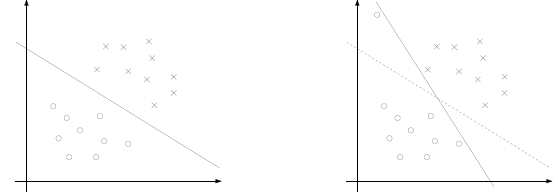当然，也存在着许多线性不可分的情况，例如下图所示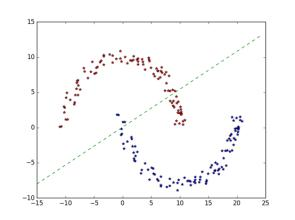即使是线性可分的，分类边界也不一定是确定的。比如下面的几个分类边界都是可以实现分类的，哪个更好呢。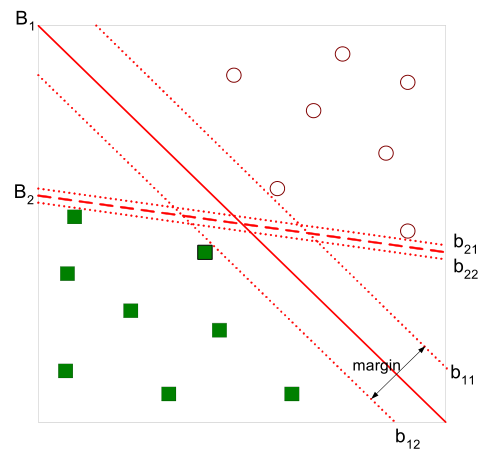这就需要用到支持向量机SVM算法了。

支持向量机/SVM

SVM（Support Vector Machines）是分类算法中应用广泛、效果不错的一类。由简至繁SVM可分类为三类：线性可分（linear SVM in linearly separable case）的线性SVM、线性不可分的线性SVM、非线性（nonlinear）SVM。

1. 线性可分

对于二类分类问题，训练集T=(x1,y1),(x2,y2),⋯,(xN,yN)$T=\left({x}_{1},{y}_{1}\right),\left({x}_{2},{y}_{2}\right),\cdots ,\left({x}_{N},{y}_{N}\right)$$T={(x_1,y_1),(x_2,y_2),⋯,(x_N,y_N)}$，其中xi${x}_{i}$$x_i$为输入样本向量，类别yi∈−1,1${y}_{i}\in -1,1$$y_i∈{-1,1}$，线性SVM通过学习得到分离超平面（hyperplane）:

w⋅x+b=0$w\cdot x+b=0$w⋅x+b=0

以及相应的分类决策函数：

f(x)=sign(w⋅x+b)$f\left(x\right)=sign\left(w\cdot x+b\right)$f(x)=sign(w⋅x+b)

其中sign$sign$$sign$为符号函数，将正数映射为1，负数映射为-1。

有如下图所示的分离超平面，哪一个超平面的分类效果更好呢？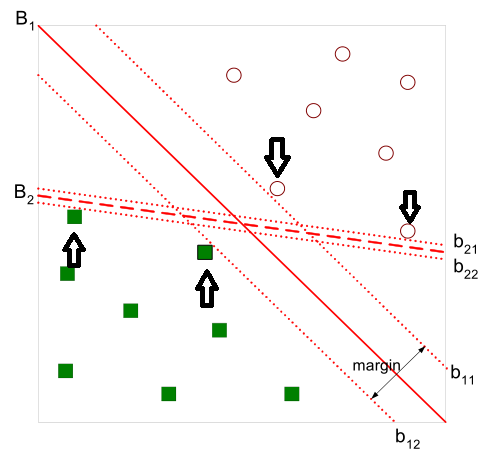直观上，超平面B1的分类效果更好一些。将距离分离超平面最近的两个不同类别的样本点称为支持向量（support vector）的，构成了两条平行于分离超平面的长带，二者之间的距离称之为margin。显然，margin更大，则分类正确的确信度更高（与超平面的距离表示分类的确信度，距离越远则分类正确的确信度越高）。通过计算得到（计算过程参考《统计学习方法》115页）

margin=2∥w∥$margin=\frac{2}{\parallel w\parallel }$margin=\frac{2}{∥w∥}

从上图中可观察到：margin以外的样本点对于确定分离超平面没有贡献，换句话说，SVM是有很重要的训练样本（支持向量）所确定的。至此，SVM分类问题可描述为在全部分类正确的情况下，最大化2∥w∥$\frac{2}{\parallel w\parallel }$$\frac{2}{∥w∥}$（等价于最小化0.5∥w∥2$0.5\parallel w{\parallel }^{2}$$0.5∥w∥^2$）；线性分类的约束最优化问题转化为如下的凸优化问题。

minw,b12||w||2$\underset{w,b}{min}\frac{1}{2}||w|{|}^{2}$\min_{w,b}\frac{1}{2}||w||^2 s.t.yi(w⋅xi+b)−1≥0$s.t.\phantom{\rule{1em}{0ex}}\phantom{\rule{1em}{0ex}}{y}_{i}\left(w\cdot {x}_{i}+b\right)-1\ge 0$s.t.\quad \quad           y_i(w\cdot x_i+b)-1 \geq 0

在线性可分情况下，训练数据集的样本点中与分离超平面距离最近的样本点的实例称为支持向量。

支持向量满足yi(w⋅xi+b)−1=0${y}_{i}\left(w\cdot {x}_{i}+b\right)-1=0$ y_i(w\cdot x_i+b)-1 =0，即正样本点满足w⋅xi+b=1$w\cdot {x}_{i}+b=1$ w\cdot x_i+b=1，负样本点满足w⋅xi+b=−1$w\cdot {x}_{i}+b=-1$ w\cdot x_i+b=-1

2. 线性支持向量机

线性可分是理想情形，大多数情况下，由于噪声或特异点等各种原因，训练样本是线性不可分的。因此，需要更一般化的学习算法。

这时我们就可以通过引入所谓的松弛变量(slack variable)，来允许有些数据点可以处于超平面的错误的一侧。这样我们的优化目标就能保持仍然不变，但是此时我们的约束条件有所改变。

凸优化问题

（a）无约束优化问题，可以写为：

minf(x)$min\phantom{\rule{1em}{0ex}}f\left(x\right)$min\quad f(x)

（b）有等式约束的优化问题，可以写为：

minf(x)s.t.hi(x)=0,i=0,1,2...n$min\phantom{\rule{1em}{0ex}}f\left(x\right)\phantom{\rule{0ex}{0ex}}s.t.\phantom{\rule{1em}{0ex}}{h}_{i}\left(x\right)=0,i=0,1,2...n$min\quad f(x)  \\

（c）有不等式约束的优化问题，可以写为：

minf(x)s.t.gi(x)≤0,i=0,1,2...nhj(x)=0,j=0,1,2...m$min\phantom{\rule{1em}{0ex}}f\left(x\right)\phantom{\rule{0ex}{0ex}}s.t.\phantom{\rule{1em}{0ex}}{g}_{i}\left(x\right)\le 0,i=0,1,2...n\phantom{\rule{0ex}{0ex}}{h}_{j}\left(x\right)=0,j=0,1,2...m$min\quad f(x)  \\
s.t. \quad g_i(x) \leq 0 ,i=0,1,2...n\\
h_j(x)=0 ,j=0,1,2...m

对于第(a)类的优化问题，尝尝使用的方法就是费马大定理(Fermat)，即使用求取函数f(x)的导数，然后令其为零，可以求得候选最优值，再在这些候选值中验证；如果是凸函数，可以保证是最优解。这也就是我们高中经常使用的求函数的极值的方法。

对于第(b)类的优化问题，常常使用的方法就是拉格朗日乘子法（Lagrange Multiplier) ，即把等式约束h_i(x)用一个系数与f(x)写为一个式子，称为拉格朗日函数，而系数称为拉格朗日乘子。通过拉格朗日函数对各个变量求导，令其为零，可以求得候选值集合，然后验证求得最优值。

对于第(c)类的优化问题，常常使用的方法就是KKT条件。同样地，我们把所有的等式、不等式约束与f(x)写为一个式子，也叫拉格朗日函数，系数也称拉格朗日乘子，通过一些条件，可以求出最优值的必要条件，这个条件称为KKT条件。

必要条件和充要条件如果不理解，可以看下面这句话：

事件M的必要条件就是M可以推出的结论
事件M的充分条件就是可以推出M的前提

了解到这些，现在让我们再看一下我们的最优化问题：

minw12||w||2s.t.yi(wTxi+b)≥1,i=0,1,2...n$\underset{w}{min}\phantom{\rule{1em}{0ex}}\frac{1}{2}||w|{|}^{2}\phantom{\rule{0ex}{0ex}}s.t.\phantom{\rule{1em}{0ex}}{y}_{i}\left({w}^{T}{x}_{i}+b\right)\ge 1,i=0,1,2...n$\min_w \quad \frac{1}{2} ||w||^2 \\
s.t. \quad y_i(w^Tx_i+b) \geq 1 ,i=0,1,2...n

现在，我们的这个对优化问题属于哪一类？很显然，它属于第(c)类问题。因为，在学习求解最优化问题之前，我们还要学习两个东西：拉格朗日函数和KKT条件。

拉格朗日函数

首先，我们先要从宏观的视野上了解一下拉格朗日对偶问题出现的原因和背景。

我们知道我们要求解的是最小化问题，所以一个直观的想法是如果我能够构造一个函数，使得该函数在可行解区域内与原目标函数完全一致，而在可行解区域外的数值非常大，甚至是无穷大，那么这个没有约束条件的新目标函数的优化问题就与原来有约束条件的原始目标函数的优化问题是等价的问题。这就是使用拉格朗日方程的目的，它将约束条件放到目标函数中，从而将有约束优化问题转换为无约束优化问题。

随后，人们又发现，使用拉格朗日获得的函数，使用求导的方法求解依然困难。进而，需要对问题再进行一次转换，即使用一个数学技巧：拉格朗日对偶。
所以，显而易见的是，我们在拉格朗日优化我们的问题这个道路上，需要进行下面二个步骤：

将有约束的原始目标函数转换为无约束的新构造的拉格朗日目标函数
使用拉格朗日对偶性，将不易求解的优化问题转化为易求解的优化

下面是拉格朗日对偶问题的公式，不想推导的记住就行了。

想看推导的参考：https://www.zhihu.com/question/58584814

我们考虑优化问题如下，记作问题（P）。

z∗=minxf(x)s.t.gi(x)≤0, ∀ i=1,…,m,x∈Xz^* = \min_x f(x)   \\
{s.t. } g_i(x)\leq 0 ,~\forall~ i=1,\ldots,m,x\in X

问题（P）的拉格朗日对偶问题（D）写作

v∗=maxα≥0minx∈Xf(x)+αTg(x)L(x,α)${v}^{\ast }=\underset{\alpha \ge 0}{max}\underset{x\in X}{min}\underset{L\left(x,\alpha \right)}{\underset{⏟}{f\left(x\right)+{\alpha }^{T}g\left(x\right)}}$v^* = \max_{α\geq 0} \min_{x\in X} \underbrace{f(x)+α^T g(x)}_{L(x,α)}

其中的函数L(x,α)就是我们熟知的拉格朗日函数。

下面，先将有约束的原始目标函数转换为无约束的新构造的拉格朗日目标函数

公式变形如下：

L(w,b,α)=12||w||2−∑i=1nαi(yi(wTxi+b)−1)$L\left(w,b,\alpha \right)=\frac{1}{2}||w|{|}^{2}-\sum _{i=1}^{n}{\alpha }_{i}\left({y}_{i}\left({w}^{T}{x}_{i}+b\right)-1\right)$ L(w,b,α) = \frac{1}{2}||w||^2-\sum_{i=1}^nα_i(y_i(w^Tx_i+b)-1)

其中αi${\alpha }_{i}$$α_i$是拉格朗日乘子，αi${\alpha }_{i}$$α_i$大于等于0，是我们构造新目标函数时引入的系数变量。

其中αi$\alpha i$$αi$是拉格朗日乘子，αi$\alpha i$$αi$大于等于0，是我们构造新目标函数时引入的系数变量(我们自己设置)。现在我们令：

θ(w)=maxαi≥0L(w,b,α)$\theta \left(w\right)=\underset{{\alpha }_{i}\ge 0}{max}L\left(w,b,\alpha \right)$\theta(w) = \max_{α_i≥0} L(w,b,α)

当样本点不满足约束条件时，即在可行解区域外：

yi(wTxi+b)<1${y}_{i}\left({w}^{T}{x}_{i}+b\right)<1$y_i(w^Tx_i+b)<1

此时，我们将αi$\alpha i$$αi$设置为正无穷，此时θ(w)$\theta \left(w\right)$$θ(w)$显然也是正无穷。

当样本点满足约束条件时，即在可行解区域内：

yi(wTxi+b)≥1${y}_{i}\left({w}^{T}{x}_{i}+b\right)\ge 1$y_i(w^Tx_i+b)≥1

此时，我们设在yi(wTxi+b)>1${y}_{i}\left({w}^{T}{x}_{i}+b\right)>1$$y_i(w^Tx_i+b)>1$时的αi$\alpha i$$αi$为0，在yi(wTxi+b)=1${y}_{i}\left({w}^{T}{x}_{i}+b\right)=1$$y_i(w^Tx_i+b)=1$时(也就是支持向量点)的αi$\alpha i$$αi$为大于0的数，那么显然θ(w)$\theta \left(w\right)$$θ(w)$为原目标函数本身。我们将上述两种情况结合一下，就得到了新的目标函数：

θ(w)={12||w||2,+∞x在可行区域x在非可行区域$\theta \left(w\right)=\left\{\begin{array}{ll}\frac{1}{2}||w|{|}^{2},& \text{x在可行区域}\\ +\mathrm{\infty }& \text{x在非可行区域}\end{array}$ θ(w) = \begin{cases} \frac{1}{2}||w||^2, & \text {x在可行区域} \\ +\infty& \text{x在非可行区域} \end{cases}

此时，再看我们的初衷，就是为了建立一个在可行解区域内与原目标函数相同，在可行解区域外函数值趋近于无穷大的新函数，现在我们做到了。

现在，我们的问题变成了求新目标函数的最小值，即：

p∗=minw,bθ(w)=minw,bmaxαi≥0L(w,b,α)${p}^{\ast }=\underset{w,b}{min}\theta \left(w\right)=\underset{w,b}{min}\underset{\alpha i\ge 0}{max}L\left(w,b,\alpha \right)$p^* = \min_{w,b}θ(w) = \min_{w,b} \max_{αi≥0}L(w,b,α)

这里用p*表示这个问题的最优值，且和最初的问题是等价的。

接下来，我们进行第二步：将不易求解的优化问题转化为易求解的优化

我们看一下我们的新目标函数，先求最大值，再求最小值。这样的话，我们首先就要面对带有需要求解的参数w和b的方程，而αi$\alpha i$$αi$又是不等式约束，这个求解过程不好做。所以，我们需要使用拉格朗日函数对偶性，将最小和最大的位置交换一下，这样就变成了：

d∗=maxα≥0minw,bL(w,b,α)${d}^{\ast }=\underset{\alpha \ge 0}{max}\underset{w,b}{min}L\left(w,b,\alpha \right)$d^* = \max_{α\geq 0} \min_{w,b} L(w,b,α)

交换以后的新问题是原始问题的对偶问题，这个新问题的最优值用d∗${d}^{\ast }$$d^*$来表示。而且d∗≤p∗${d}^{\ast }\le {p}^{\ast }$$d^*≤p^*$。我们关心的是d=p$d=p$$d=p$的时候，这才是我们要的解。需要什么条件才能让d=p$d=p$$d=p$呢？

首先必须满足这个优化问题是凸优化问题。
其次，需要满足KKT条件。

凸优化问题的定义是：求取最小值的目标函数为凸函数的一类优化问题。目标函数是凸函数我们已经知道，这个优化问题又是求最小值。所以我们的最优化问题就是凸优化问题。

接下里，就是探讨是否满足KKT条件了。

KKT条件

我们已经使用拉格朗日函数对我们的目标函数进行了处理，生成了一个新的目标函数。通过一些条件，可以求出最优值的必要条件，这个条件就是接下来要说的KKT条件。一个最优化模型能够表示成下列标准形式：

minf(x)s.t.hj(x)=0,j=1,2,3...p,s.t.gk(x)≤0,k=1,2,....,q,x∈X⊂Rn$minf\left(x\right)\phantom{\rule{0ex}{0ex}}s.t.\phantom{\rule{thinmathspace}{0ex}}\phantom{\rule{thinmathspace}{0ex}}\phantom{\rule{thinmathspace}{0ex}}{h}_{j}\left(x\right)=0,\phantom{\rule{thinmathspace}{0ex}}\phantom{\rule{thinmathspace}{0ex}}\phantom{\rule{thinmathspace}{0ex}}\phantom{\rule{thinmathspace}{0ex}}\phantom{\rule{thinmathspace}{0ex}}\phantom{\rule{thinmathspace}{0ex}}j=1,2,3...p,\phantom{\rule{0ex}{0ex}}s.t.\phantom{\rule{thinmathspace}{0ex}}\phantom{\rule{thinmathspace}{0ex}}\phantom{\rule{thinmathspace}{0ex}}\phantom{\rule{thinmathspace}{0ex}}{g}_{k}\left(x\right)\le 0,k=1,2,....,q,\phantom{\rule{0ex}{0ex}}x\in X\subset {R}^{n}$\min f(x)   \\
s.t. \,\,\, h_j(x)=0,  \,\,\,\,\,\,   j=1,2,3...p, \\
s.t.   \,\,\,\, g_k(x)\leq 0 ,k=1,2,....,q,  \\
x \in X \subset R^n

优化模型的拉格朗日函数为

L(X,λ,μ)=f(X)+∑j=1pλjhj(X)+∑k=1qμkgk(X)$L\left(X,\lambda ,\mu \right)=f\left(X\right)+\sum _{j=1}^{p}{\lambda }_{j}{h}_{j}\left(X\right)+\sum _{k=1}^{q}{\mu }_{k}{g}_{k}\left(X\right)$L(X,λ,μ)=f(X)+\sum_{j=1}^p λ_jh_j(X)+\sum_{k=1}^qμ_kg_k(X)

其中f(x)$f\left(x\right)$$f(x)$是原目标函数，hj(x)${h}_{j}\left(x\right)$$h_j(x)$是第j$j$$j$个等式约束条件，λj${\lambda }_{j}$$λ_j$是对应的约束系数，gk${g}_{k}$$g_k$是不等式约束，uk${u}_{k}$$u_k$是对应的约束系数。

KKT条件的全称是Karush-Kuhn-Tucker条件，KKT条件是说最优值条件必须满足以下条件：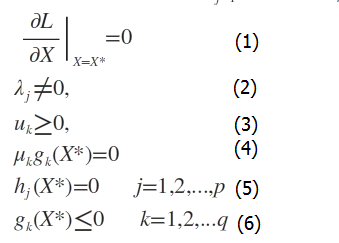其中X∗${X}^{\ast }$$X^*$表示样本点。这些求解条件就是KKT条件。(1)是对拉格朗日函数取极值时候带来的一个必要条件，(2)是拉格朗日系数约束（同等式情况），(3)是不等式约束情况，(4)是互补松弛条件，(5)、(6)是原约束条件。

对于一般的任意问题而言，KKT条件是使一组解成为最优解的必要条件，当原问题是凸问题的时候，KKT条件也是充分条件。

对于我们的优化问题：

minw12||w||2s.t.yi(wTxi+b)≥1,i=0,1,2...n$\underset{w}{min}\phantom{\rule{1em}{0ex}}\frac{1}{2}||w|{|}^{2}\phantom{\rule{0ex}{0ex}}s.t.\phantom{\rule{1em}{0ex}}{y}_{i}\left({w}^{T}{x}_{i}+b\right)\ge 1,i=0,1,2...n$\min_w \quad \frac{1}{2} ||w||^2 \\
s.t. \quad y_i(w^Tx_i+b) \geq 1 ,i=0,1,2...n

显然，条件二已经满足了。另外两个条件为啥也满足呢？

这里原谅我省略一系列证明步骤，感兴趣的可以移步这里：点我查看

这里已经给出了很好的解释。现在，凸优化问题和KKT都满足了，问题转换成了对偶问题。而求解这个对偶学习问题，可以分为三个步骤：首先要让L(w,b,α)关于w和b最小化，然后求对α的极大，最后利用SMO算法求解对偶问题中的拉格朗日乘子。现在，我们继续推导。

对偶问题求解

第一步：根据上述推导已知：

L(w,b,α)=12||w||2−∑i=1nαi(yi(wTxi+b)−1)$L\left(w,b,\alpha \right)=\frac{1}{2}||w|{|}^{2}-\sum _{i=1}^{n}{\alpha }_{i}\left({y}_{i}\left({w}^{T}{x}_{i}+b\right)-1\right)$ L(w,b,α) = \frac{1}{2}||w||^2-\sum_{i=1}^nα_i(y_i(w^Tx_i+b)-1)

首先固定α$\alpha$$α$，要让L(w,b,α)$L\left(w,b,\alpha \right)$$L(w,b,α)$关于w$w$$w$和b$b$$b$最小化，我们分别对w$w$$w$和b$b$$b$偏导数，令其等于0，即：

dLdw=0⟹w=∑i=1nαiyixi$\frac{dL}{dw}=0\phantom{\rule{thickmathspace}{0ex}}⟹\phantom{\rule{thickmathspace}{0ex}}w=\sum _{i=1}^{n}{\alpha }_{i}{y}_{i}{x}_{i}$ \frac{d L}{d w} = 0 \implies w = \sum_{i=1}^n α_iy_ix_i
dLdb=0⟹∑i=1nαiyi=0$\frac{dL}{db}=0\phantom{\rule{thickmathspace}{0ex}}⟹\phantom{\rule{thickmathspace}{0ex}}\sum _{i=1}^{n}{\alpha }_{i}{y}_{i}=0$ \frac{d L}{d b} = 0 \implies  \sum_{i=1}^n α_iy_i=0

将上述结果带回L(w,b,α)$L\left(w,b,\alpha \right)$$L(w,b,α)$得到

L(w,b,α)=∑i=1nαi−0.5∗∑i,j=1nαiαjyiyjxTixj$L\left(w,b,\alpha \right)=\sum _{i=1}^{n}{\alpha }_{i}-0.5\ast \sum _{i,j=1}^{n}{\alpha }_{i}{\alpha }_{j}{y}_{i}{y}_{j}{x}_{i}^{T}{x}_{j}$L(w,b,α) = \sum_{i=1}^n α_i-0.5*\sum_{i,j=1}^n α_iα_jy_iy_jx_i^Tx_j

从上面的最后一个式子，我们可以看出，此时的L(w,b,α)$L\left(w,b,\alpha \right)$$L(w,b,α)$函数只含有一个变量，即αi${\alpha }_{i}$$α_i$。

第二步：

现在内侧的最小值求解完成，我们求解外侧的最大值，从上面的式子得到

maxα∑i=1nαi−12∑i,j=1nαiαjyiyjxTixjs.t.αi≥0,i=1,2...n,∑i=1nαiyi=0$\underset{\alpha }{max}\sum _{i=1}^{n}{\alpha }_{i}-\frac{1}{2}\sum _{i,j=1}^{n}{\alpha }_{i}{\alpha }_{j}{y}_{i}{y}_{j}{x}_{i}^{T}{x}_{j}\phantom{\rule{0ex}{0ex}}s.t.\phantom{\rule{thinmathspace}{0ex}}\phantom{\rule{thinmathspace}{0ex}}\phantom{\rule{thinmathspace}{0ex}}\phantom{\rule{thinmathspace}{0ex}}{\alpha }_{i}\ge 0,i=1,2...n,\phantom{\rule{0ex}{0ex}}\sum _{i=1}^{n}{\alpha }_{i}{y}_{i}=0$\max_α\sum_{i=1}^n α_i-\frac{1}{2}\sum_{i,j=1}^n α_iα_jy_iy_jx_i^Tx_j \\
s.t. \,\,\,\,  α_i\geq0,i=1,2...n,  \\
\sum_{i=1}^n α_iy_i=0

现在我们的优化问题变成了如上的形式。对于这个问题，我们有更高效的优化算法，即序列最小优化（SMO）算法。我们通过这个优化算法能得到α，再根据α，我们就可以求解出w和b，进而求得我们最初的目的：找到超平面，即”决策平面”。

而上式可以等价于
minα12∑i,j=1nαiαjyiyjxTixj−∑i=1nαis.t.αi≥0,i=1,2...n,∑i=1nαiyi=0$\underset{\alpha }{min}\frac{1}{2}\sum _{i,j=1}^{n}{\alpha }_{i}{\alpha }_{j}{y}_{i}{y}_{j}{x}_{i}^{T}{x}_{j}-\sum _{i=1}^{n}{\alpha }_{i}\phantom{\rule{0ex}{0ex}}s.t.\phantom{\rule{thinmathspace}{0ex}}\phantom{\rule{thinmathspace}{0ex}}\phantom{\rule{thinmathspace}{0ex}}\phantom{\rule{thinmathspace}{0ex}}{\alpha }_{i}\ge 0,i=1,2...n,\phantom{\rule{0ex}{0ex}}\sum _{i=1}^{n}{\alpha }_{i}{y}_{i}=0$ \min_α \frac{1}{2}\sum_{i,j=1}^n α_iα_jy_iy_jx_i^Tx_j  -\sum_{i=1}^n α_i\\
s.t. \,\,\,\,  α_i\geq0,i=1,2...n,  \\
\sum_{i=1}^n α_iy_i=0

总结一句话：我们为啥使出吃奶的劲儿进行推导？因为我们要将最初的原始问题，转换到可以使用SMO算法求解的问题，这是一种最流行的求解方法。

SMO算法

SMO算法的目标是求出一系列αi${\alpha }_{i}$$α_i$和b$b$$b$，一旦求出了这些αi${\alpha }_{i}$$α_i$，就很容易计算出权重向量w并得到分隔超平面。

SMO算法的工作原理是：每次循环中选择两个αi${\alpha }_{i}$$α_i$进行优化处理。一旦找到了一对合适的αi${\alpha }_{i}$$α_i$，那么就增大其中一个同时减小另一个。这里所谓的”合适”就是指两个αi${\alpha }_{i}$$α_i$必须符合以下两个条件，条件之一就是两个αi${\alpha }_{i}$$α_i$必须要在间隔边界之外，而且第二个条件则是这两个αi${\alpha }_{i}$$α_i$还没有进行过区间化处理或者不在边界上。

SMO算法的解法

先来定义特征到结果的输出函数为：

u=wTx+b$u={w}^{T}x+b$u=w^Tx+b
接着，我们回忆一下原始优化问题，如下：

minw12||w||2s.t.yi(wTxi+b)≥1,i=0,1,2...n$\underset{w}{min}\phantom{\rule{1em}{0ex}}\frac{1}{2}||w|{|}^{2}\phantom{\rule{0ex}{0ex}}s.t.\phantom{\rule{1em}{0ex}}{y}_{i}\left({w}^{T}{x}_{i}+b\right)\ge 1,i=0,1,2...n$\min_w \quad \frac{1}{2} ||w||^2 \\
s.t. \quad y_i(w^Tx_i+b) \geq 1 ,i=0,1,2...n

求导得：

w=∑i=1nαiyixi$w=\sum _{i=1}^{n}{\alpha }_{i}{y}_{i}{x}_{i}$w = \sum_{i=1}^n α_iy_ix_i
将上述公式带入输出函数中：

u=∑i=1nαiyixTix+b$u=\sum _{i=1}^{n}{\alpha }_{i}{y}_{i}{x}_{i}^{T}x+b$u = \sum_{i=1}^n α_iy_ix_i^Tx+b

与此同时，拉格朗日对偶后得到最终的目标化函数：

minα12∑i,j=1nαiαjyiyjxTixj−∑i=1nαis.t.αi≥0,i=1,2...n,∑i=1nαiyi=0$\underset{\alpha }{min}\frac{1}{2}\sum _{i,j=1}^{n}{\alpha }_{i}{\alpha }_{j}{y}_{i}{y}_{j}{x}_{i}^{T}{x}_{j}-\sum _{i=1}^{n}{\alpha }_{i}\phantom{\rule{0ex}{0ex}}s.t.\phantom{\rule{thinmathspace}{0ex}}\phantom{\rule{thinmathspace}{0ex}}\phantom{\rule{thinmathspace}{0ex}}\phantom{\rule{thinmathspace}{0ex}}{\alpha }_{i}\ge 0,i=1,2...n,\phantom{\rule{0ex}{0ex}}\sum _{i=1}^{n}{\alpha }_{i}{y}_{i}=0$ \min_α \frac{1}{2}\sum_{i,j=1}^n α_iα_jy_iy_jx_i^Tx_j  -\sum_{i=1}^n α_i\\
s.t. \,\,\,\,  α_i\geq0,i=1,2...n,  \\
\sum_{i=1}^n α_iy_i=0

线性支持下的SMO

实际上，对于上述目标函数，是存在一个假设的，即数据100%线性可分。但是，目前为止，我们知道几乎所有数据都不那么”干净”。这时我们就可以通过引入所谓的松弛变量(slack variable)，来允许有些数据点可以处于超平面的错误的一侧。这样我们的优化目标就能保持仍然不变，但是此时我们的约束条件有所改变：

s.t.C≥αi≥0,i=1,2...n,∑i=1nαiyi=0$s.t.\phantom{\rule{1em}{0ex}}C\ge {\alpha }_{i}\ge 0,i=1,2...n,\phantom{\rule{0ex}{0ex}}\sum _{i=1}^{n}{\alpha }_{i}{y}_{i}=0$ s.t.\quad C\geqα_i\geq0,i=1,2...n,  \\
\sum_{i=1}^n α_iy_i=0

根据KKT条件可以得出其中αi$\alpha i$$αi$取值的意义为：

αi=0⟺yiui≥1${\alpha }_{i}=0\phantom{\rule{thickmathspace}{0ex}}⟺\phantom{\rule{thickmathspace}{0ex}}{y}_{i}{u}_{i}\ge 1$α_i = 0  \iff  y_iu_i\geq1
0<αi<C⟺yiui=1$0<{\alpha }_{i}0<α_i <C \iff  y_iu_i=1
αi=C⟺yiui≤1${\alpha }_{i}=C\phantom{\rule{thickmathspace}{0ex}}⟺\phantom{\rule{thickmathspace}{0ex}}{y}_{i}{u}_{i}\le 1$α_i = C  \iff  y_iu_i\leq1

对于第1种情况，表明αi$\alpha i$$αi$是正常分类，在边界内部；
对于第2种情况，表明αi$\alpha i$$αi$是支持向量，在边界上；
对于第3种情况，表明αi$\alpha i$$αi$是在两条边界之间。

而最优解需要满足KKT条件，即上述3个条件都得满足，以下几种情况出现将会不满足

yiui≤1αi<C${y}_{i}{u}_{i}\le 1\phantom{\rule{1em}{0ex}}{\alpha }_{i}y_iu_i\leq1   \quad  α_i <C
yiui≥1αi>0${y}_{i}{u}_{i}\ge 1\phantom{\rule{1em}{0ex}}{\alpha }_{i}>0$y_iu_i\geq1 \quad  α_i > 0
yiui=1αi=0或者αi=C${y}_{i}{u}_{i}=1\phantom{\rule{1em}{0ex}}{\alpha }_{i}=0或者{\alpha }_{i}=C$ y_iu_i=1   \quad   α_i = 0 或者 α_i =C

也就是说，如果存在不能满足KKT条件的αi$\alpha i$$αi$，那么需要更新这些αi$\alpha i$$αi$，这是第一个约束条件。此外，更新的同时还要受到第二个约束条件的限制，即：

∑i=1nαiyi=0$\sum _{i=1}^{n}{\alpha }_{i}{y}_{i}=0$\sum_{i=1}^n α_iy_i=0

因为这个条件，我们同时更新两个α$\alpha$$α$值，因为只有成对更新，才能保证更新之后的值仍然满足和为0的约束，假设我们选择的两个乘子为α1${\alpha }_{1}$$α_1$和α2${\alpha }_{2}$$α_2$：
αnew1y1+αnew2y2=αold1y1+αold2y2=ζ${\alpha }_{1}^{new}{y}_{1}+{\alpha }_{2}^{new}{y}_{2}={\alpha }_{1}^{old}{y}_{1}+{\alpha }_{2}^{old}{y}_{2}=\zeta$α_1^{new}y_1+α_2^{new}y_2 = α_1^{old}y_1+α_2^{old}y_2 = \zeta

其中， ζ$\zeta$$\zeta$为常数。因为两个因子不好同时求解，所以可以先求第二个乘子α2${\alpha }_{2}$$α_2$的解(αnew2)$\left({\alpha }_{2}^{new}\right)$$(α_2^{new})$，得到α2${\alpha }_{2}$$α_2$的解(αnew2)$\left({\alpha }_{2}^{new}\right)$$(α_2^{new})$之后，再用α2${\alpha }_{2}$$α_2$的解(αnew2)$\left({\alpha }_{2}^{new}\right)$$(α_2^{new})$表示α1${\alpha }_{1}$$α_1$的解(αnew1)$\left({\alpha }_{1}^{new}\right)$$(α_1^{new})$。为了求解(αnew2)$\left({\alpha }_{2}^{new}\right)$$(α_2^{new})$ ，得先确定(αnew2)$\left({\alpha }_{2}^{new}\right)$$(α_2^{new})$的取值范围。假设它的上下边界分别为H和L，那么有：

L≤αnew2≤H$L\le {\alpha }_{2}^{new}\le H$L\leqα_2^{new}\leq H

接下来，综合下面两个条件：

C≥αi≥0,i=1,2...n,αnew1y1+αnew2y2=αold1y1+αold2y2=ζ$C\ge {\alpha }_{i}\ge 0,i=1,2...n,\phantom{\rule{0ex}{0ex}}{\alpha }_{1}^{new}{y}_{1}+{\alpha }_{2}^{new}{y}_{2}={\alpha }_{1}^{old}{y}_{1}+{\alpha }_{2}^{old}{y}_{2}=\zeta$ C\geqα_i\geq0,i=1,2...n,  \\
α_1^{new}y_1+α_2^{new}y_2 = α_1^{old}y_1+α_2^{old}y_2 = \zeta

当y1不等于y2时，即一个为正1，一个为负1的时候，可以得到：

αold1−αold2=ζ${\alpha }_{1}^{old}-{\alpha }_{2}^{old}=\zeta$α_1^{old}-α_2^{old}= \zeta

所以有：

L=max(0,ζ),H=min(C,C−ζ)$L=max\left(0,\zeta \right),H=min\left(C,C-\zeta \right)$L=max(0, \zeta),H=min(C,C-\zeta)

此时，取值范围如下图所示：当y1等于y2时，即两个都为正1或者都为负1，可以得到：

αold1+αold2=ζ${\alpha }_{1}^{old}+{\alpha }_{2}^{old}=\zeta$α_1^{old}+α_2^{old}= \zeta

所以有：

L=max(0,ζ−C),H=min(C,ζ)$L=max\left(0,\zeta -C\right),H=min\left(C,\zeta \right)$L=max(0, \zeta-C),H=min(C,\zeta)

此时，取值范围如下图所示：如此，根据y1和y2异号或同号，可以得出αnew2${\alpha }_{2}^{new}$$α_2^{new}$的上下界分别为：
L=max(0,αold2−αold1),H=min(C,C+αold2−αold1)ify1≠y2$L=max\left(0,{\alpha }_{2}^{old}-{\alpha }_{1}^{old}\right),H=min\left(C,C+{\alpha }_{2}^{old}-{\alpha }_{1}^{old}\right)\phantom{\rule{2em}{0ex}}if\phantom{\rule{1em}{0ex}}{y}_{1}\ne {y}_{2}$L=max(0, α_2^{old}-α_1^{old}),H=min(C,C+α_2^{old}-α_1^{old})  \qquad if \quad  y_1 \neq y_2
L=max(0,αold1+αold2−C),H=min(C,αold1+αold2)ify1=y2$L=max\left(0,{\alpha }_{1}^{old}+{\alpha }_{2}^{old}-C\right),H=min\left(C,{\alpha }_{1}^{old}+{\alpha }_{2}^{old}\right)\phantom{\rule{2em}{0ex}}if\phantom{\rule{1em}{0ex}}{y}_{1}={y}_{2}$L=max(0, α_1^{old}+α_2^{old}-C),H=min(C,α_1^{old}+α_2^{old})  \qquad if \quad  y_1=y_2

这个界限就是编程的时候需要用到的。已经确定了边界，接下来，就是推导迭代式，用于更新 α$\alpha$$α$值。

我们已经知道，更新α$\alpha$$α$的边界，接下来就是讨论如何更新α$\alpha$$α$值。我们依然假设选择的两个乘子为α1${\alpha }_{1}$$α_1$和α2${\alpha }_{2}$$α_2$。(推导略)

αnew,clipped2=⎧⎩⎨⎪⎪H,αnew2,L,αnew2>HL≤αnew2≤Hαnew2<L${\alpha }_{2}^{new,clipped}=\left\{\begin{array}{ll}H,& {\alpha }_{2}^{new}>H\\ {\alpha }_{2}^{new},& L\le {\alpha }_{2}^{new}\le H\\ L,& {\alpha }_{2}^{new} α_2^{new,clipped}= \begin{cases} H, & α_2^{new}>H \\  α_2^{new}, & L\leq α_2^{new}\leq H  \\ L, & α_2^{new}<L \end{cases}

αnew1=αold1+y1y2(αold2−αnew,clipped2)${\alpha }_{1}^{new}={\alpha }_{1}^{old}+{y}_{1}{y}_{2}\left({\alpha }_{2}^{old}-{\alpha }_{2}^{new,clipped}\right)$ α_1^{new}= α_1^{old}+y_1y_2(α_2^{old}-α_2^{new,clipped})

这样，我们就知道了怎样计算α1${\alpha }_{1}$$α_1$和α2${\alpha }_{2}$$α_2$了，也就是如何对选择的α进行更新。

我们要根据α$\alpha$$α$ 的取值范围，去更正b的值，使间隔最大化。当αnew1${\alpha }_{1}^{new}$$α_1^{new}$在0和C之间的时候，根据KKT条件可知，这个点是支持向量上的点。因此，满足下列公式：

y1(wTx1+b)=1${y}_{1}\left({w}^{T}{x}_{1}+b\right)=1$ y_1(w^Tx_1+b)= 1

公式两边同时乘以y1${y}_{1}$$y_1$得(y1×y1=1${y}_{1}×{y}_{1}=1$$y_1\times y_1=1$)：

∑i=1nαiyixix1+b=y1$\sum _{i=1}^{n}{\alpha }_{i}{y}_{i}{x}_{i}{x}_{1}+b={y}_{1}$  \sum_{i=1}^nα_iy_ix_ix_1+b=y_1

因为我们是根据α1${\alpha }_{1}$$α_1$和α2${\alpha }_{2}$$α_2$的值去更新b，所以单独提出i=1和i=2的时候，整理可得：

bnew1=y1−∑i=3nαiyixTix1−αnew1y1xT1x1−αnew2y2xT2x1${b}_{1}^{new}={y}_{1}-\sum _{i=3}^{n}{\alpha }_{i}{y}_{i}{x}_{i}^{T}{x}_{1}-{\alpha }_{1}^{new}{y}_{1}{x}_{1}^{T}{x}_{1}-{\alpha }_{2}^{new}{y}_{2}{x}_{2}^{T}{x}_{1}$  b_1^{new} = y_1-\sum_{i=3}^nα_iy_ix_i^Tx_1-α_1^{new}y_1x_1^Tx_1-α_2^{new}y_2x_2^Tx_1

其中前两项为：

y1−∑i=3nαiyixTix1=−E1+αold1y1xT1x1+αold2y2xT2x1+bold${y}_{1}-\sum _{i=3}^{n}{\alpha }_{i}{y}_{i}{x}_{i}^{T}{x}_{1}=-{E}_{1}+{\alpha }_{1}^{old}{y}_{1}{x}_{1}^{T}{x}_{1}+{\alpha }_{2}^{old}{y}_{2}{x}_{2}^{T}{x}_{1}+{b}^{old}$y_1-\sum_{i=3}^nα_iy_ix_i^Tx_1=-E_1+α_1^{old}y_1x_1^Tx_1+α_2^{old}y_2x_2^Tx_1+b^{old}

将上述两个公式，整理得：

bnew1=bold−E1−y1(αnew1−αold1)xT1x1−y2(αnew2−αold2)xT2x1${b}_{1}^{new}={b}^{old}-{E}_{1}-{y}_{1}\left({\alpha }_{1}^{new}-{\alpha }_{1}^{old}\right){x}_{1}^{T}{x}_{1}-{y}_{2}\left({\alpha }_{2}^{new}-{\alpha }_{2}^{old}\right){x}_{2}^{T}{x}_{1}$  b_1^{new} =b^{old}-E_1-y_1(α_1^{new}-α_1^{old})x_1^Tx_1-y_2(α_2^{new}-α_2^{old})x_2^Tx_1

同理可得bnew2${b}_{2}^{new}$$b_2^{new}$为：

bnew2=bold−E2−y1(αnew1−αold1)xT1x2−y2(αnew2−αold2)xT2x2${b}_{2}^{new}={b}^{old}-{E}_{2}-{y}_{1}\left({\alpha }_{1}^{new}-{\alpha }_{1}^{old}\right){x}_{1}^{T}{x}_{2}-{y}_{2}\left({\alpha }_{2}^{new}-{\alpha }_{2}^{old}\right){x}_{2}^{T}{x}_{2}$  b_2^{new} =b^{old}-E_2-y_1(α_1^{new}-α_1^{old})x_1^Tx_2-y_2(α_2^{new}-α_2^{old})x_2^Tx_2

当我们更新了α1${\alpha }_{1}$$α_1$和α2${\alpha }_{2}$$α_2$之后，需要重新计算阈值b，因为b关系到了我们f(x)$f\left(x\right)$$f(x)$的计算，也就关系到了误差Ei的计算。

b=⎧⎩⎨⎪⎪b1,b2,(b1+b2)/2,0<αnew1<C0<αnew2<C≤Hotherwise$b=\left\{\begin{array}{ll}{b}_{1},& 0<{\alpha }_{1}^{new} b= \begin{cases} b_1, & 0<α_1^{new}<C \\  b_2, & 0<α_2^{new}<C \leq H  \\ (b_1+b_2)/2, & otherwise \end{cases}

现在，让我们梳理下SMO算法的步骤：

步骤1：计算误差：

Ei=f(xi)−yi=∑j=1nαjyjxTixj+b−yi${E}_{i}=f\left({x}_{i}\right)-{y}_{i}=\sum _{j=1}^{n}{\alpha }_{j}{y}_{j}{x}_{i}^{T}{x}_{j}+b-{y}_{i}$E_i=f(x_i)-y_i=\sum_{j=1}^nα_jy_jx_i^Tx_j+b-y_i

步骤2：计算上下界L和H：

L=max(0,αoldj−αoldi),H=min(C,C+αoldj−αoldi)ifyi≠yj$L=max\left(0,{\alpha }_{j}^{old}-{\alpha }_{i}^{old}\right),H=min\left(C,C+{\alpha }_{j}^{old}-{\alpha }_{i}^{old}\right)\phantom{\rule{2em}{0ex}}if\phantom{\rule{1em}{0ex}}{y}_{i}\ne {y}_{j}$L=max(0, α_j^{old}-α_i^{old}),H=min(C,C+α_j^{old}-α_i^{old})  \qquad if \quad  y_i \neq y_j
L=max(0,αoldj+αoldi−C),H=min(C,αoldj+αoldi)ifyj=yi$L=max\left(0,{\alpha }_{j}^{old}+{\alpha }_{i}^{old}-C\right),H=min\left(C,{\alpha }_{j}^{old}+{\alpha }_{i}^{old}\right)\phantom{\rule{2em}{0ex}}if\phantom{\rule{1em}{0ex}}{y}_{j}={y}_{i}$L=max(0, α_j^{old}+α_i^{old}-C),H=min(C,α_j^{old}+α_i^{old})  \qquad if \quad  y_j=y_i

步骤3：计算η$\eta$$η$：

η=xTixi+xTjxj−2xTixj$\eta ={x}_{i}^{T}{x}_{i}+{x}_{j}^{T}{x}_{j}-2{x}_{i}^{T}{x}_{j}$η = x_i^Tx_i+x_j^Tx_j-2x_i^Tx_j

步骤4：更新αj${\alpha }_{j}$$α_j$：

αnewj=αoldj+yj(Ei−Ej)η${\alpha }_{j}^{new}={\alpha }_{j}^{old}+\frac{{y}_{j}\left({E}_{i}-{E}_{j}\right)}{\eta }$α_j^{new}=α_j^{old}+\frac{y_j(E_i-E_j)}{η }

步骤5：根据取值范围修剪αj${\alpha }_{j}$$α_j$

αnew,clippedj=⎧⎩⎨⎪⎪H,αnewj,L,αnewj>HL≤αnewj≤Hαnewj<L${\alpha }_{j}^{new,clipped}=\left\{\begin{array}{ll}H,& {\alpha }_{j}^{new}>H\\ {\alpha }_{j}^{new},& L\le {\alpha }_{j}^{new}\le H\\ L,& {\alpha }_{j}^{new} α_j^{new,clipped}= \begin{cases} H, & α_j^{new}>H \\  α_j^{new}, & L\leq α_j^{new}\leq H  \\ L, & α_j^{new}<L \end{cases}

步骤6：更新αi${\alpha }_{i}$$α_i$：

αnewi=αoldi+yiyj(αoldj−αnew,clippedj)${\alpha }_{i}^{new}={\alpha }_{i}^{old}+{y}_{i}{y}_{j}\left({\alpha }_{j}^{old}-{\alpha }_{j}^{new,clipped}\right)$ α_i^{new}= α_i^{old}+y_iy_j(α_j^{old}-α_j^{new,clipped})

步骤7：更新b1和b2：

bnew1=bold−Ei−yi(αnewi−αoldi)xTixi−yj(αnewj−αoldj)xTjxi${b}_{1}^{new}={b}^{old}-{E}_{i}-{y}_{i}\left({\alpha }_{i}^{new}-{\alpha }_{i}^{old}\right){x}_{i}^{T}{x}_{i}-{y}_{j}\left({\alpha }_{j}^{new}-{\alpha }_{j}^{old}\right){x}_{j}^{T}{x}_{i}$  b_1^{new} =b^{old}-E_i-y_i(α_i^{new}-α_i^{old})x_i^Tx_i-y_j(α_j^{new}-α_j^{old})x_j^Tx_i

bnew2=bold−Ej−yi(αnewi−αoldi)xTixj−yj(αnewj−αoldj)xTjxj${b}_{2}^{new}={b}^{old}-{E}_{j}-{y}_{i}\left({\alpha }_{i}^{new}-{\alpha }_{i}^{old}\right){x}_{i}^{T}{x}_{j}-{y}_{j}\left({\alpha }_{j}^{new}-{\alpha }_{j}^{old}\right){x}_{j}^{T}{x}_{j}$  b_2^{new} =b^{old}-E_j-y_i(α_i^{new}-α_i^{old})x_i^Tx_j-y_j(α_j^{new}-α_j^{old})x_j^Tx_j

步骤8：根据b1和b2更新b：

b=⎧⎩⎨⎪⎪b1,b2,(b1+b2)/2,0<αnew1<C0<αnew2<C≤Hotherwise$b=\left\{\begin{array}{ll}{b}_{1},& 0<{\alpha }_{1}^{new} b= \begin{cases} b_1, & 0<α_1^{new}<C \\  b_2, & 0<α_2^{new}<C \leq H  \\ (b_1+b_2)/2, & otherwise \end{cases}

python实现

样本集下载：https://github.com/626626cdllp/data-mining/blob/master/SVM/testSet.txt

# -*- coding:UTF-8 -*-
import matplotlib.pyplot as plt
import numpy as np
import random

# 简化版smo

# 函数说明:读取数据
dataMat = alldata[:,0:2]   #添加数据
labelMat = alldata[:,2]   #.astype(int).reshape(-1,1)  #添加标签
return dataMat,labelMat

"""
函数说明:随机选择alpha

Parameters:
i - alpha_i的索引值
m - alpha参数个数
Returns:
j - alpha_j的索引值

"""
def selectJrand(i, m):
j = i                                 #选择一个不等于i的j
while (j == i):
j = int(random.uniform(0, m))
return j

"""
函数说明:修剪alpha

Parameters:
aj - alpha_j值
H - alpha上限
L - alpha下限
Returns:
aj - alpah值

"""
def clipAlpha(aj,H,L):
if aj > H:
aj = H
if L > aj:
aj = L
return aj

# 函数说明:数据可视化
def showDataSet(dataMat, labelMat):

place_plus = np.where(labelMat==1)   # 正样本的位置
place_minus = np.where(labelMat==-1)  # 负样本的位置
data_plus = dataMat[place_plus]    #正样本
data_minus = dataMat[place_minus]  #负样本

plt.scatter(np.transpose(data_plus), np.transpose(data_plus))   #正样本散点图
plt.scatter(np.transpose(data_minus), np.transpose(data_minus)) #负样本散点图
plt.show()

"""
函数说明:简化版SMO算法

Parameters:
dataMatIn - 数据矩阵
classLabels - 数据标签
C - 松弛变量
toler - 容错率
maxIter - 最大迭代次数
"""
def smoSimple(dataMatIn, classLabels, C, toler, maxIter):
#转换为numpy的mat存储
dataMatrix = np.mat(dataMatIn)
labelMat = np.mat(classLabels).transpose()
#初始化b参数，统计dataMatrix的维度
b = 0; m,n = np.shape(dataMatrix)
#初始化alpha参数，设为0
alphas = np.mat(np.zeros((m,1)))
#初始化迭代次数
iter_num = 0
#最多迭代matIter次
while (iter_num < maxIter):
alphaPairsChanged = 0
for i in range(m):
#步骤1：计算误差Ei
fXi = float(np.multiply(alphas,labelMat).T*(dataMatrix*dataMatrix[i,:].T)) + b
Ei = fXi - float(labelMat[i])
#优化alpha，设定一定的容错率。
if ((labelMat[i]*Ei < -toler) and (alphas[i] < C)) or ((labelMat[i]*Ei > toler) and (alphas[i] > 0)):
#随机选择另一个与alpha_i成对优化的alpha_j
j = selectJrand(i,m)
#步骤1：计算误差Ej
fXj = float(np.multiply(alphas,labelMat).T*(dataMatrix*dataMatrix[j,:].T)) + b
Ej = fXj - float(labelMat[j])
#保存更新前的aplpha值，使用深拷贝
alphaIold = alphas[i].copy(); alphaJold = alphas[j].copy();
#步骤2：计算上下界L和H
if (labelMat[i] != labelMat[j]):
L = max(0, alphas[j] - alphas[i])
H = min(C, C + alphas[j] - alphas[i])
else:
L = max(0, alphas[j] + alphas[i] - C)
H = min(C, alphas[j] + alphas[i])
if L==H: print("L==H"); continue
#步骤3：计算eta
eta = 2.0 * dataMatrix[i,:]*dataMatrix[j,:].T - dataMatrix[i,:]*dataMatrix[i,:].T - dataMatrix[j,:]*dataMatrix[j,:].T
if eta >= 0: print("eta>=0"); continue
#步骤4：更新alpha_j
alphas[j] -= labelMat[j]*(Ei - Ej)/eta
#步骤5：修剪alpha_j
alphas[j] = clipAlpha(alphas[j],H,L)
if (abs(alphas[j] - alphaJold) < 0.00001): print("alpha_j变化太小"); continue
#步骤6：更新alpha_i
alphas[i] += labelMat[j]*labelMat[i]*(alphaJold - alphas[j])
#步骤7：更新b_1和b_2
b1 = b - Ei- labelMat[i]*(alphas[i]-alphaIold)*dataMatrix[i,:]*dataMatrix[i,:].T - labelMat[j]*(alphas[j]-alphaJold)*dataMatrix[i,:]*dataMatrix[j,:].T
b2 = b - Ej- labelMat[i]*(alphas[i]-alphaIold)*dataMatrix[i,:]*dataMatrix[j,:].T - labelMat[j]*(alphas[j]-alphaJold)*dataMatrix[j,:]*dataMatrix[j,:].T
#步骤8：根据b_1和b_2更新b
if (0 < alphas[i]) and (C > alphas[i]): b = b1
elif (0 < alphas[j]) and (C > alphas[j]): b = b2
else: b = (b1 + b2)/2.0
#统计优化次数
alphaPairsChanged += 1
#打印统计信息
print("第%d次迭代 样本:%d, alpha优化次数:%d" % (iter_num,i,alphaPairsChanged))
#更新迭代次数
if (alphaPairsChanged == 0): iter_num += 1
else: iter_num = 0
print("迭代次数: %d" % iter_num)
return b,alphas

"""
函数说明:分类结果可视化

Parameters:
dataMat - 数据矩阵
w - 直线法向量
b - 直线解决
"""
def showClassifer(dataMat, w, b):
# 绘制样本点
place_plus = np.where(labelMat==1)   # 正样本的位置
place_minus = np.where(labelMat==-1)  # 负样本的位置

data_plus = dataMat[place_plus]    #正样本
data_minus = dataMat[place_minus]  #负样本

plt.scatter(np.transpose(data_plus), np.transpose(data_plus),s=30, alpha=0.7)   #正样本散点图
plt.scatter(np.transpose(data_minus), np.transpose(data_minus), s=30, alpha=0.7) #负样本散点图

#绘制直线
x1 = max(dataMat[:,0])  # 第一个属性的最大值
x2 = min(dataMat[:,0])  # 第一个属性的最小值
a1, a2 = w
b = float(b)
a1 = float(a1)
a2 = float(a2)
y1, y2 = (-b- a1*x1)/a2, (-b - a1*x2)/a2
plt.plot([x1, x2], [y1, y2])
#找出支持向量点
for i, alpha in enumerate(alphas):
if abs(alpha) > 0:
x, y = dataMat[i]
plt.scatter([x], [y], s=150, c='none', alpha=0.7, linewidth=1.5, edgecolor='red')
plt.show()

"""
函数说明:计算w

Parameters:
dataMat - 数据矩阵
labelMat - 数据标签
alphas - alphas值
"""
def get_w(dataMat, labelMat, alphas):
alphas, dataMat, labelMat = np.array(alphas), np.array(dataMat), np.array(labelMat)
w = np.dot((np.tile(labelMat.reshape(1, -1).T, (1, 2)) * dataMat).T, alphas)
return w.tolist()

if __name__ == '__main__':
showDataSet(dataMat,labelMat)
b,alphas = smoSimple(dataMat, labelMat, 0.6, 0.001, 40)
w = get_w(dataMat, labelMat, alphas)
showClassifer(dataMat, w, b)

3、非线性向量机

1 核技巧

我们已经了解到，SVM如何处理线性可分的情况，而对于非线性的情况，SVM的处理方式就是选择一个核函数。简而言之：在线性不可分的情况下，SVM通过某种事先选择的非线性映射（核函数）将输入变量映到一个高维特征空间，将其变成在高维空间线性可分，在这个高维空间中构造最优分类超平面。

线性可分的情况下，可知最终的超平面方程为：
f(x)=∑i=1nαiyixTix+b$f\left(x\right)=\sum _{i=1}^{n}{\alpha }_{i}{y}_{i}{x}_{i}^{T}x+b$f(x)=\sum_{i=1}^nα_iy_ix_i^Tx+b

将上述公式用内积来表示：
f(x)=∑i=1nαiyi<xi,x>+b$f\left(x\right)=\sum _{i=1}^{n}{\alpha }_{i}{y}_{i}<{x}_{i},x>+b$f(x)=\sum_{i=1}^nα_iy_i<x_i,x>+b

对于线性不可分，我们使用一个非线性映射，将数据映射到特征空间，在特征空间中使用线性学习器，分类函数变形如下：
f(x)=∑i=1nαiyi<ϕ(xi),ϕ(x)>+b$f\left(x\right)=\sum _{i=1}^{n}{\alpha }_{i}{y}_{i}<\varphi \left({x}_{i}\right),\varphi \left(x\right)>+b$f(x)=\sum_{i=1}^nα_iy_i<ϕ(x_i),ϕ(x)>+b

其中ϕ$\varphi$$ϕ$从输入空间(X)到某个特征空间(F)的映射，这意味着建立非线性学习器分为两步：

首先使用一个非线性映射将数据变换到一个特征空间F；
然后在特征空间使用线性学习器分类。

如果有一种方法可以在特征空间中直接计算内积<ϕ(xi),ϕ(x)>$<\varphi \left({x}_{i}\right),\varphi \left(x\right)>$$<ϕ(x_i),ϕ(x)>$，就像在原始输入点的函数中一样，就有可能将两个步骤融合到一起建立一个分线性的学习器，这样直接计算的方法称为核函数方法。

这里直接给出一个定义：核是一个函数k，对所有x,z∈X$x,z\in X$$x,z∈X$，满足k(x,z)=<ϕ(xi),ϕ(x)>$k\left(x,z\right)=<\varphi \left({x}_{i}\right),\varphi \left(x\right)>$$k(x,z)=<ϕ(x_i),ϕ(x)>$，这里ϕ(⋅)$\varphi \left(·\right)$$ϕ(·)$是从原始输入空间X到内积空间F的映射。

简而言之：如果不是用核技术，就会先计算线性映ϕ(x1)和ϕ(x2)$\varphi \left({x}_{1}\right)和\varphi \left({x}_{2}\right)$$ϕ(x_1)和ϕ(x_2)$，然后计算这它们的内积，使用了核技术之后，先把ϕ(x1)$\varphi \left({x}_{1}\right)$$ϕ(x_1)$和ϕ(x2)$\varphi \left({x}_{2}\right)$$ϕ(x_2)$的一般表达式<ϕ(x1),ϕ(x2)>=k(<ϕ(x1),ϕ(x2)>)$<\varphi \left({x}_{1}\right),\varphi \left({x}_{2}\right)>=k\left(<\varphi \left({x}_{1}\right),\varphi \left({x}_{2}\right)>\right)$$<ϕ(x_1),ϕ(x_2)>=k(<ϕ(x_1),ϕ(x_2) >)$计算出来，这里的<⋅，⋅>$<·，·>$$<·，·>$表示内积，k(⋅，⋅)$k\left(·，·\right)$$k(·，·)$就是对应的核函数，这个表达式往往非常简单，所以计算非常方便。

这种将内积替换成核函数的方式被称为核技巧(kernel trick)。

核函数的数学要求

核函数有严格的数学要求，所以设计一个核函数是很困难的。K(x,z)是正定核的充要条件是：K（x,z）对应的Gram矩阵实半正定矩阵。

Gram矩阵：矩阵对应点的内积。KTK, KKT

半正定矩阵：设A是实对称矩阵。如果对任意的实非零列矩阵X有XTAX≥0，就称A为半正定矩阵。

当检验一个K是否为正定核函数，要对任意有限输入集{xi…}验证K对应的Gram矩阵实是否为半正定矩阵。

LIBSVM中提供的核函数

线性核函数：
K(x,z)=x⋅z$K\left(x,z\right)=x\cdot z$K(x,z)=x\cdot z
线性核，主要用于线性可分的情况，我们可以看到特征空间到输入空间的维度是一样的，其参数少速度快，对于线性可分数据，其分类效果很理想，因此我们通常首先尝试用线性核函数来做分类，看看效果如何，如果不行再换别的

多项式核函数：
K(x,z)=(x⋅z+1)p$K\left(x,z\right)=\left(x\cdot z+1{\right)}^{p}$K(x,z)=(x\cdot z+1)^p
对应的支持向量机为p次多项式分类器。
多项式核函数可以实现将低维的输入空间映射到高纬的特征空间，但是多项式核函数的参数多，当多项式的阶数比较高的时候，核矩阵的元素值将趋于无穷大或者无穷小，计算复杂度会大到无法计算。

RBF核函数（高斯核函数） ：
K(x,z)=exp(−||x−z||2